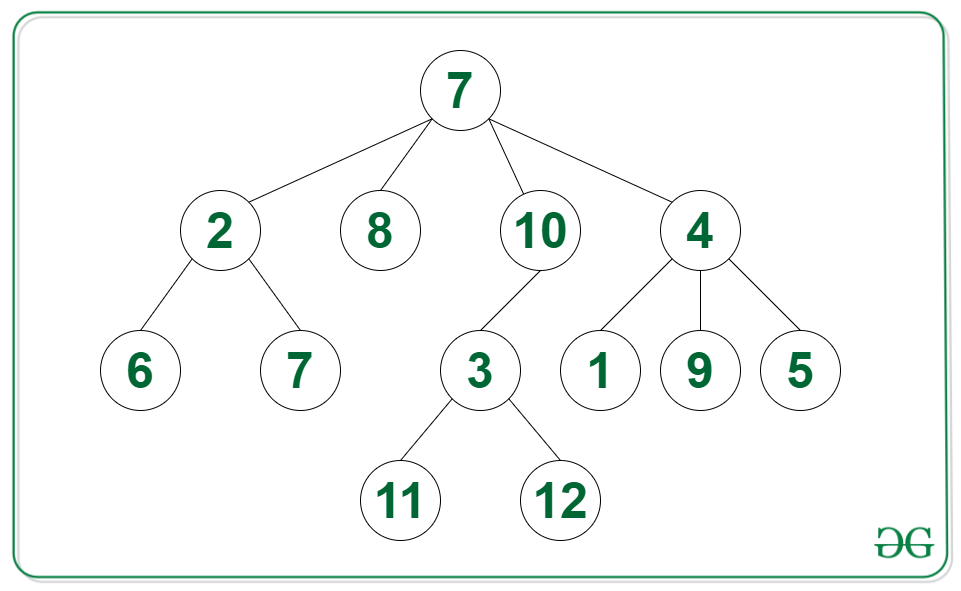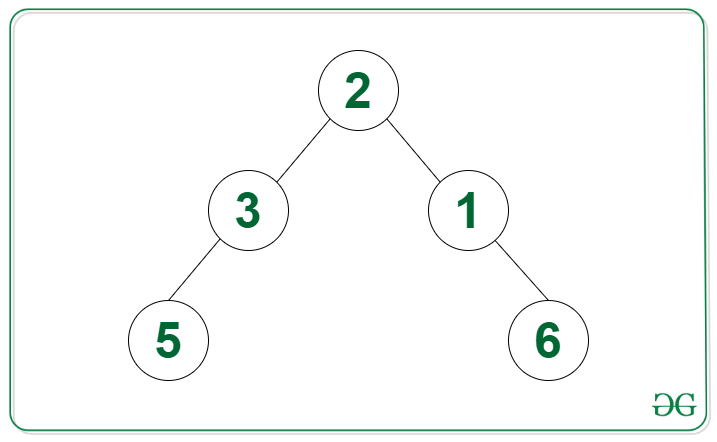Count of nodes in a given N-ary tree having distance to all leaf nodes equal in their subtree

• Last Updated : 11 Jan, 2022

Given an N-ary tree root, the task is to find the number of non-leaf nodes in the tree such that all the leaf nodes in the subtree of the current node are at an equal distance from the current node.

Example:

Input: Tree in the below image
Output: 4
Explanation: The nodes {10, 3, 2, 4} have the distance between them and all the leaf nodes in their subtree respectively as equal.Input: Tree in the image below
Output: 3Approach: The given problem can be solved by using the post-order traversal. The idea is to check if the number of nodes from the current node to all its leaf nodes is the same. Below steps can be followed to solve the problem:

• Apply post-order traversal on the N-ary tree:
• If the root has no children then return 1 to the parent
• If every branch has equal height then increment the count by 1 and return height + 1 to the parent
• Or else return -1 to the parent indicating that the branches have unequal height
• Return the count as the answer

C++

 // C++ code for the above approach #include using namespace std; class Node { public:     vector children;     int val;     // constructor     Node(int v)     {         val = v;         children = {};     } };   // Post-order traversal to find // depth of all branches of every // node of the tree int postOrder(Node* root, int count[]) {         // If root is a leaf node     // then return 1     if (root->children.size() == 0)         return 1;         // Initialize a variable height     // calculate longest increasing path     int height = 0;         // Use recursion on all child nodes     for (Node* child : root->children)     {                 // Get the height of the branch         int h = postOrder(child, count);                 // Initialize height of first         // explored branch         if (height == 0)             height = h;                 // If branches are unbalanced         // then store -1 in height         else if (h == -1 || height != h)             height = -1;     }         // Increment the value of count     // If height is not -1     if (height != -1)         count++;         // Return the height of branches     // including the root if height is     // not -1 or else return -1     return height != -1 ? height + 1 : -1; }   // Function to find the number of nodes // in the N-ary tree with their branches // having equal height int equalHeightBranches(Node* root) {         // Base case     if (root == NULL)         return 0;         // Initialize a variable count     // to store the answer     int count = { 0 };         // Apply post order traversal     // on the tree     postOrder(root, count);         // Return the answer     return count; } // Driver code int main() {     // Initialize the tree     Node* seven = new Node(7);     Node* seven2 = new Node(7);     Node* five = new Node(5);     Node* four = new Node(4);     Node* nine = new Node(9);     Node* one = new Node(1);     Node* two = new Node(2);     Node* six = new Node(6);     Node* eight = new Node(8);     Node* ten = new Node(10);     Node* three = new Node(3);     Node* mfour = new Node(-4);     Node* mtwo = new Node(-2);     Node* zero = new Node(0);     three->children.push_back(mfour);     three->children.push_back(mtwo);     three->children.push_back(zero);     ten->children.push_back(three);     two->children.push_back(six);     two->children.push_back(seven2);     four->children.push_back(nine);     four->children.push_back(one);     four->children.push_back(five);     seven->children.push_back(ten);     seven->children.push_back(two);     seven->children.push_back(eight);     seven->children.push_back(four);         // Call the function     // and print the result     cout << (equalHeightBranches(seven)); }   // This code is contributed by Potta Lokesh

Python3

 # Python code for the above approach class Node:     def __init__(self, val):         self.val = val         self.children = []   # Post-order traversal to find # depth of all branches of every # node of the tree def postOrder(root, count):           # If root is a leaf node         # then return 1     if (len(root.children) == 0):         return 1       # Initialize a variable height     # calculate longest increasing path     height = 0       # Use recursion on all child nodes     for child in root.children:           # Get the height of the branch         h = postOrder(child, count)           # Initialize height of first         # explored branch         if (height == 0):             height = h           # If branches are unbalanced         # then store -1 in height         elif (h == -1 or height != h):             height = -1       # Increment the value of count     #  If height is not -1     if (height != -1):         count += 1       # Return the height of branches     # including the root if height is     # not -1 or else return -1     if(height != -1):         return height + 1     else:         return -1   # Function to find the number of nodes # in the N-ary tree with their branches # having equal height def equalHeightBranches(root):       # Base case     if (root == None):         return 0       # Initialize a variable count     # to store the answer     count =        # Apply post order traversal     # on the tree     postOrder(root, count)       # Return the answer     return count   # Driver code   # Initialize the tree seven = Node(7) seven2 = Node(7) five = Node(5) four = Node(4) nine = Node(9) one = Node(1) two = Node(2) six = Node(6) eight = Node(8) ten = Node(10) three = Node(3) mfour = Node(-4) mtwo = Node(-2) zero = Node(0) three.children.append(mfour) three.children.append(mtwo) three.children.append(zero) ten.children.append(three) two.children.append(six) two.children.append(seven2) four.children.append(nine) four.children.append(one) four.children.append(five) seven.children.append(ten) seven.children.append(two) seven.children.append(eight) seven.children.append(four)   # Call the function # and print the result print(equalHeightBranches(seven))   # This code is contributed by rj13to.

Javascript



Output

4

Time Complexity: O(N)
Auxiliary Space: O(H), where H is the height of the tree

My Personal Notes arrow_drop_up
Recommended Articles
Page :